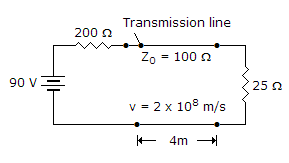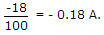Electronics and Communication Engineering - Microwave Communication - Discussion

7.

In the given figure the reflected current wave after first reflection is[A]. 0.18 A [B]. -0.18 A [C]. 0.3 A [D]. -0.3 A

Explanation:Anonymous said: (Sep 29, 2015) How -18 comes here?

 Ankur said: (Feb 21, 2017) -18? How? Explain.

 Ahir said: (Mar 31, 2018) -18 is a reflected voltage.

 Amir said: (Aug 8, 2018) Where does -18 come from? Explain.

 Ebenezer Ajaying said: (Sep 23, 2018) Reflection coefficient at the source=1/3; Reflection coefficient at the load= -3/5; Therefore, the reflection voltage wave after the first reflection = Vs* source reflection coefficient* load reflection coefficient. = 90*1/3*3/5 = -18V.